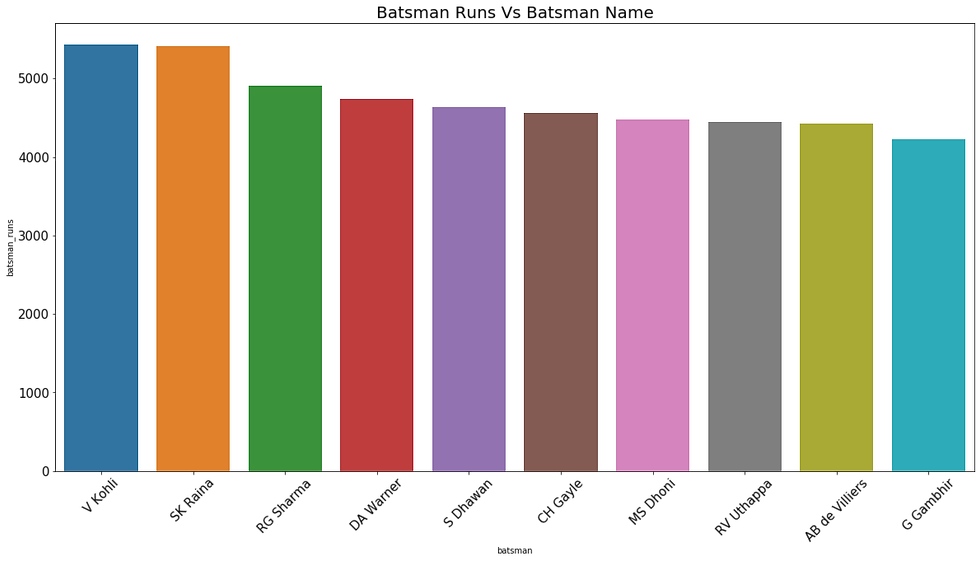top of page
Search

# INDIAN PREMIER LEAGUE DATA ANALYSISIn this Article we are going to analyze the IPL dataset. In this analysis we are focusing on bowler performance during the Indian premier league. The data which has been used in this article has been gathered from Kaggle. The data set consists of data about IPL matches played from the 2008 to 2019. We have two dataset deliveries and matches. In Deliveries data set contains the 21 attributes and 179078 records and The matches dataset contains 18 attributes and 756 records.

Our Objective

To find top 10 Player names who takes most wickets

To find top 10 Player names who have bowled most no balls

To find top 10 Player names who have bowled most wide balls

To find top 10 Player names by their bowling average

To find top 10 Player names by their bowling strike rate

To find top 10 Player names by their bowling Economy rate

Number of winning teams

To find top 10 Player names who most runs

To find top 10 Player names who become the most of the time man of the match

Our Goal :

• Basic Exploratory Analysis

• Features Analysis

Dependencies/Libraries Required:

In this step, we imported all the required libraries like seaborn, pandas(for preprocessing), math, matplotlib etc.

```import pandas as pd
import numpy as np
import matplotlib.pyplot as plt
import seaborn as sns
import math
from IPython.display import display  ```

```ipl_deliveries = 'IPL Data 2008 to 2019\\deliveries.csv'
ipl_match = 'IPL Data 2008 to 2019\\matches.csv'

Output :

Displaying deliveries datasetAttributes names of deliveries datasetMatches dataAttributes name of matches datasetData Preparation and Data Cleaning

The data set found inaccurate records. the same team with two different names. So In this step, replace the team name and venue name also. Now the data is ready for analysis.

Code snippet :

```ipl_match.team1.replace({'Rising Pune Supergiants' : 'Rising Pune Supergiant'},regex=True,inplace=True)
ipl_match.team2.replace({'Rising Pune Supergiants' : 'Rising Pune Supergiant'},regex=True,inplace=True)
ipl_match.winner.replace({'Rising Pune Supergiants' : 'Rising Pune Supergiant'},regex=True,inplace=True)
ipl_match.venue.replace({'Feroz Shah Kotla Ground':'Feroz Shah Kotla',

Now here first we replace the name of id to match_id and then combined the data by their match_id ```

Code Snippet :

```ipl_match.rename(columns={'id':'match_id'},inplace=True)
combine_data = pd.merge(ipl_deliveries,ipl_match,on='match_id')
pd.set_option('display.max_columns',None)

Now In this step, Gathered information about the bowler and then stored it into a dictionary.

Code Snippet :

```bowler_performance={}
for i in range(0, len(combine_data['bowler'])):
try:
total_balls = bowler_performance[combine_data['bowler'][i]] + 1
total_runs = bowler_performance[combine_data['bowler'][i]] + combine_data['total_runs'][i]
total_wickets = bowler_performance[combine_data['bowler'][i]]
wide_balls = bowler_performance[combine_data['bowler'][i]]
no_balls = bowler_performance[combine_data['bowler'][i]]
if(combine_data['wide_runs'][i] != 0):
wide_balls = wide_balls + 1
if(combine_data['noball_runs'][i] != 0):
no_balls = no_balls + 1
try:
if(math.isnan(combine_data['dismissal_kind'][i])):
bowler_performance[combine_data['bowler'][i]] = [total_balls, total_runs, total_wickets, wide_balls, no_balls]
except:
total_wickets = bowler_performance[combine_data['bowler'][i]] + 1
bowler_performance[combine_data['bowler'][i]] = [total_balls, total_runs, total_wickets, wide_balls, no_balls]
except:
try:
if(math.isnan(combine_data['dismissal_kind'][i])):
bowler_performance[combine_data['bowler'][i]] = [0, combine_data['total_runs'][i], 1, 0, 0 ]
except:
bowler_performance[combine_data['bowler'][i]] = [0, combine_data['total_runs'][i], 0, 0, 0 ]
analysis_condition = []
analysis_condition.append(['Name', 'Total balls', 'Total runs', 'Total wickets','Wide balls', 'No balls'])
for i in bowler_performance:
analysis_condition.append([[i], bowler_performance[i], bowler_performance[i], bowler_performance[i], bowler_performance[i], bowler_performance[i]])
print(analysis_condition)```

Output :In this step we extract the information from the dictionary using a for loop and store it into a list after create a dataframe and store it all data from the list.

Here To visualize the data defined a function to plot the bar plot

Code Snippet :

```def bar_plot(data,x,y,titles):
plt.figure(figsize=(20,10))
sns.barplot(x, y, data=data[:10])
plt.title(titles,size=20)
plt.xticks(rotation=45,size=15)
plt.yticks(size=15)
plt.show()```

Calling the bar_plot function to visualize the top 10 Player names who take most wickets. In the Barplot we can see the SL malinga takes the most wickets in IPL session from 2008 to 2019

Code snippet :

```tw = bowler_data[:].sort_values(by='Total_wickets',ascending=False)
bar_plot(tw,'Bowler_name','Total_wickets','Bowler Names vs Total Wickets')```Call the defined function to visualize the top 10 Player names who have bowled most wide balls. In the Barplot we can see the SL malinga bowled the most wide ball in IPL session from 2008 to 2019

Code Snippet :

```twb=bowler_data[:].sort_values(by='Total_wide_balls',ascending=False)
bar_plot(twb,'Bowler_name','Total_wide_balls','Bowler Names vs Total Wide Balls')```Call the defined function to visualize the top 10 Player names who have bowled most No balls. In the Barplot we can see the S Sreesanth bowled the most No balls in IPL session from 2008 to 2019

Code snippet :

```tnb=bowler_data[:].sort_values(by='Total_No_balls',ascending=False)
bar_plot(tnb,'Bowler_name','Total_No_balls','Bowler Names vs Total No Balls')```Call the defined function to visualize the top 10 Player names Highest bowling average. In the Barplot we can see the K Goel is the number one position highest bowling average in IPL session from 2008 to 2019

Code snippet :

```tba=bowler_data[:].sort_values(by='Bowling_average',ascending=False)
bar_plot(tba,'Bowler_name','Bowling_average','Bowler Names vs Bowling_average')```Call the defined function to visualize the top 10 Player names Highest bowling strike rate. In the Barplot we can see the K Goel is the number one position highest bowling strike rate in IPL session from 2008 to 2019

Code snippet :

```tsr=bowler_data[:].sort_values(by='Strike_rate',ascending=False)
bar_plot(tsr,'Bowler_name','Strike_rate','Bowler Names vs Top Strike Rate')```Call the defined function to visualize the top 10 Player names Highest bowling Economy rate. In the Barplot we can see the K Goel is the number one position highest bowling Economy rate in IPL session from 2008 to 2019

Code snippet :

```ter=bowler_data[:].sort_values(by='Economy_rate',ascending=False)
bar_plot(ter,'Bowler_name','Economy_rate','Bowler Names vs Top Economy Rate')```We can see the graph most of the times Mumbai indians win the match in all IPL seasons and at the second and third position is chennai super kings and kolkata knight riders.

Code snippet :

```plt.figure(figsize=(20,10))
ax = sns.countplot(x="winner", data=ipl_match)
ax.set_title("Number of matches win",size = 15);
plt.xticks(rotation=45,size=15);
ax.set_xlabel('Teams',size = 15);
ax.set_ylabel('Number of occurences',size = 15);
plt.show()```We can see in the graph that Virat kohli is in first position in making the highest runs.

Code snippet :

```batsman_data = ipl_deliveries.groupby(['batsman']).sum().reset_index()
best_batsman=batsman_data[:].sort_values(by='batsman_runs',ascending=False)
bar_plot(best_batsman,'batsman','batsman_runs','Batsman Runs Vs Batsman Name')```We can see in the graph that Chris Gayle is in first position for most of the time man of the match and at the second position is AB de Villiers.

Code snippet :

```plt.figure(figsize=(20,10))
ax = sns.countplot(x="player_of_match", data=ipl_match ,order = ipl_match['player_of_match'].value_counts().index[:20:1])
ax.set_title("Top players Become man of the match",size = 15);
plt.xticks(rotation=45,size=15);
ax.set_xlabel('Teams',size = 15);
ax.set_ylabel('Number of occurences',size = 15);
plt.show()```Thank You